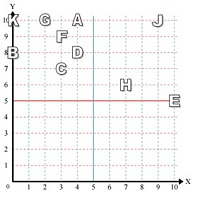# Reading a point in quadrant 1 Online Quiz

#### Complete Python Prime Pack

9 Courses     2 eBooks

#### Artificial Intelligence & Machine Learning Prime Pack

6 Courses     1 eBooks

#### Java Prime Pack

9 Courses     2 eBooks

Following quiz provides Multiple Choice Questions (MCQs) related to Reading a point in quadrant 1. You will have to read all the given answers and click over the correct answer. If you are not sure about the answer then you can check the answer using Show Answer button. You can use Next Quiz button to check new set of questions in the quiz.Q 1 - Use the grid below to determine the coordinates where letter J is located.### Explanation

The x coordinate of the letter J is 9 and The y coordinate is 10.

So the coordinates of the letter J are (9, 10)

Q 2 - Use the grid below to determine the coordinates where letter F is located.### Explanation

The x coordinate of the letter F is 3 and The y coordinate is 9.

So the coordinates of the letter ‘F’ are (3, 9)

Q 3 - Use the grid below to determine the coordinates where letter K is located.### Explanation

The x coordinate of the letter K is 0 and The y coordinate is 10.

So the coordinates of the letter K are (0, 10)

Q 4 - Use the grid below to determine the coordinates where letter C is located.### Explanation

The x coordinate of the letter C is 3 and The y coordinate is 7.

So the coordinates of the letter ‘C’ are (3, 7)

Q 5 - Use the grid below to determine the coordinates where letter A is located.### Explanation

The x coordinate of the letter A is 4 and The y coordinate is 10.

So the coordinates of the letter A are (4, 10)

Q 6 - Use the grid below to determine the coordinates where letter B is located.### Explanation

The x coordinate of the letter B is 0 and The y coordinate is 8.

So the coordinates of the letter ‘B’ are (0, 8)

Q 7 - Use the grid below to determine the coordinates where letter D is located.### Explanation

The x coordinate of the letter D is 4 and The y coordinate is 8.

So the coordinates of the letter ‘D’ are (4, 8)

Q 8 - Use the grid below to determine the coordinates where letter E is located.### Explanation

The x coordinate of the letter E is 10 and The y coordinate is 5.

So the coordinates of the letter E are (10, 5)

Q 9 - Use the grid below to determine the coordinates where letter H is located.### Explanation

The x coordinate of the letter H is 7 and The y coordinate is 6.

So the coordinates of the letter ‘H’ are (7, 6)

Q 10 - Use the grid below to determine the coordinates where letter G is located.### Explanation

The x coordinate of the letter G is 2 and The y coordinate is 10.

So the coordinates of the letter G are (2, 10)